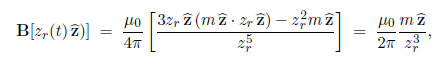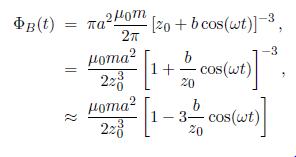Mathematics

# Electrodynamics and Conservation Laws: Mathematical Explanation

A magnetic dipole of moment M = mz is located at the origin. A thin circular conducting ring of radius a  vibrates such that the position of its centre is r = [z0 + b cos (ωt)]z with b << a < 20. The plane of the ring remains parallel to the x—y plane during the vibration. Find the emf around the ring in the φ direction.

So, The magnetic field of the dipole is

B (r) = μ0 / 4π [ {3r (m.r) – r2m} / r5]

Since b << a < z0 we can approximate the magnetic field anywhere on the ring as it vibrates byWhere, zr(t) = [z0 + b cos (ωt)]z, is the height of the ring.

The magnetic flux through the loop is:Since a << z0. Hence,

Ɛ = – (dφB / dt)

So, Ɛ ≈ – [ 3μ0ma2bω / 2z04] sin (ωt)